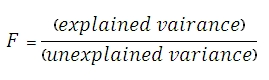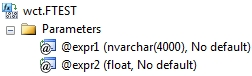# SQL Server F.TEST function

FTEST

Updated: 28 February 2011

Note: This documentation is for the SQL2008 (and later) version of this XLeratorDB function, it is not compatible with SQL Server 2005.
Click here for the SQL2005 version of the FTEST function

Use the aggregate function FTEST to return the result of an F-test. An F-test returns the two-tailed probability that the variance in dataset 1 and dataset 2 are not significantly different. Use this function to determine whether two samples have different variances.SyntaxArguments
@GroupName
the name, as text, associated with @Value. There can only be 2 labels in a group.
@Value
the value to be used in the F-test calculation. @Value is an expression of type float or of a type that can be implicitly converted to float.
Return Types
float
Remarks
·         If the number of rows in dataset 1 or dataset 2 is less than 2, FTEST will return an error
·         If the variance of dataset 1 or the variance of dataset 2 is zero, FTEST will return a divide by zero error.
·         FTEST is designed for normalized data.
·         If there are more than the 2 datasets in the group, FTEST will return a NULL.
·         FTEST is an aggregate function and follows the same conventions as all other aggregate functions in SQL Server.
·         If you have previously used the FTEST scalar function, the FTEST aggregate has a different syntax. The FTEST scalar function is no longer available in XLeratorDB/statistics2008, though you can still use the scalar FTEST_q
Examples
SELECT wct.FTEST(
label       --@GroupName
,x          --@Value
) as FTEST
FROM (VALUES
('d1', 6),('d1', 7),('d1', 9),('d1', 15),('d1', 21),
('d2', 20),('d2', 28),('d2', 31),('d2', 38),('d2', 40)
) n(label, x)

This produces the following result
FTEST
----------------------
0.648317846786174

(1 row(s) affected)

If the values are not normalized, we could try something like this.
SELECT wct.FTEST(
label
,x
) as FTEST
FROM (VALUES
(6,20),(7,28),(9,31),(15,38),(21,40)
) n(d1, d2)
CROSS APPLY(VALUES
('d1', d1),
('d2', d2)
) f(label, x)

This produces the following result
FTEST
----------------------
0.648317846786174

(1 row(s) affected)

Here’s an example, where the 2 arrays are passed as in 2 rows in a spreadsheet format.
SELECT wct.FTEST(n.label, f.x) as FTEST
FROM (VALUES
('d1',6,7,9,15,21),
('d2',20,28,31,38,40)
) n(label,x1,x2,x3,x4,x5)
CROSS APPLY(VALUES
('x1', x1),
('x2', x2),
('x3', x3),
('x4', x4),
('x5', x5)
) f(v, x)

This produces the following result.
FTEST
----------------------
0.648317846786174

(1 row(s) affected)

In this example, we have two trials with 2 datasets each. We can use the GROUP BY syntax to do the F-test calculation on both trials
simultaneously.
SELECT trial
,wct.FTEST(dataset, val) as FTEST
FROM (VALUES
('Trial 1','d1',93.2923),
('Trial 1','d1',101.7884),
('Trial 1','d1',105.3499),
('Trial 1','d1',101.7043),
('Trial 1','d1',91.0345),
('Trial 1','d1',74.2144),
('Trial 1','d1',102.1458),
('Trial 1','d1',88.6409),
('Trial 1','d1',93.2016),
('Trial 1','d1',115.4339),
('Trial 1','d2',107.6562),
('Trial 1','d2',74.2587),
('Trial 1','d2',100.7526),
('Trial 1','d2',91.3729),
('Trial 1','d2',104.4295),
('Trial 1','d2',91.3057),
('Trial 1','d2',104.412),
('Trial 1','d2',92.2344),
('Trial 1','d2',108.6575),
('Trial 1','d2',88.7054),
('Trial 2','d3',109.5777),
('Trial 2','d3',110.0512),
('Trial 2','d3',82.1003),
('Trial 2','d3',94.4187),
('Trial 2','d3',96.8142),
('Trial 2','d3',100.3303),
('Trial 2','d3',100.7579),
('Trial 2','d3',70.4202),
('Trial 2','d3',99.1351),
('Trial 2','d3',82.6996),
('Trial 2','d4',89.3049),
('Trial 2','d4',121.954),
('Trial 2','d4',91.2342),
('Trial 2','d4',77.2809),
('Trial 2','d4',95.6451),
('Trial 2','d4',85.728),
('Trial 2','d4',87.8381),
('Trial 2','d4',71.1317),
('Trial 2','d4',121.6113),
('Trial 2','d4',126.1566)
) n(trial, dataset, val)
GROUP BY Trial

This produces the following result.
trial                    FTEST
------- ----------------------
Trial 1      0.903558917831079
Trial 2      0.211079812120368

(2 row(s) affected)

### SupportCopyright 2008-2019 Westclintech LLC         Privacy Policy        Terms of Service